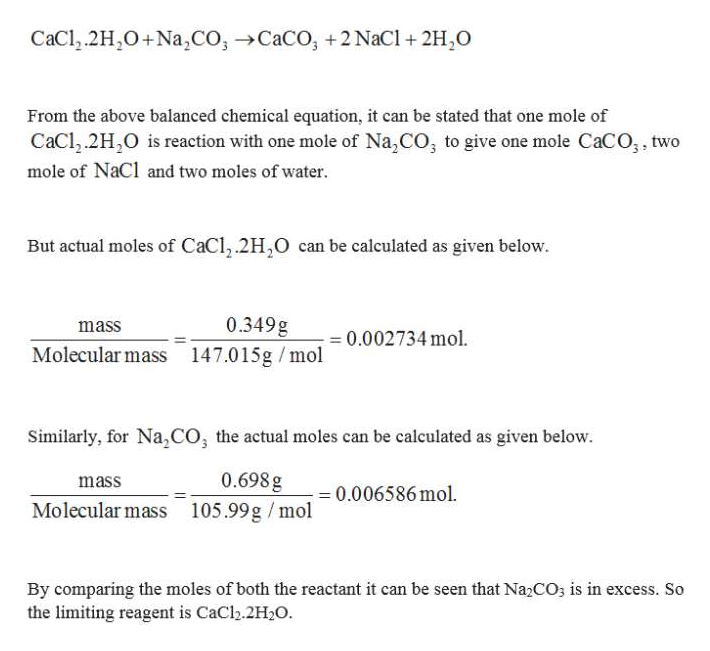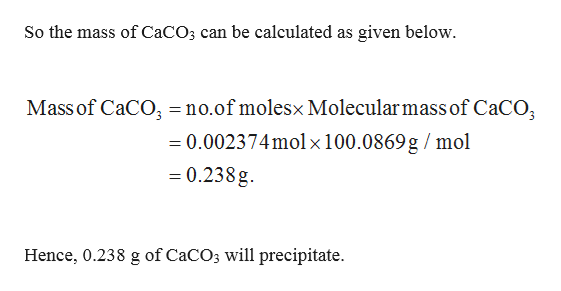# 0.349 g of CaCl2•2H2O and 0.698 g of Na2CO3 are dissolved in 100 mL of water to forma solution.a. What is the limiting reactant?b. How many grams of CaCO3 will precipitate?c. How many grams of the excess reactant remain after the reaction has gone tocompletion?

Question
3 views

0.349 g of CaCl2•2H2O and 0.698 g of Na2CO3 are dissolved in 100 mL of water to form
a solution.
a. What is the limiting reactant?
b. How many grams of CaCO3 will precipitate?
c. How many grams of the excess reactant remain after the reaction has gone to
completion?

check_circle

Step 1

(a) The resulting equation is given below.help_outlineImage TranscriptioncloseCaCl.2H2O+Na,CO,CaCO, +2 NaCl +2H,O From the above balanced chemical equation, it can be stated that one mole of CaCl.2H,O is reaction with one mole of Na,CO, to give one mole CaCO;, two mole of NaCl and two moles of water But actual moles of CaCl,.2H2O can be calculated as given below 0.349g mass - 0.002734 mol. Molecular mass 147.015g /mol Similarly, for Na,cO, the actual moles can be calculated as given below. 0.698 g mass 0.006586 mol. Molecular mass 105.99g /mol By comparing the moles of both the reactant it can be seen that Na2CO3 is in excess. So the limiting reagent is CaCl.2H2O. fullscreen
Step 2

(b) 0.002734 mol of CaCl2.2H2O will produce 0.002734 mol of CaCO3 according...help_outlineImage TranscriptioncloseSo the mass of CaCO3 can be calculated as given below Mass of CaCO3 =no.of molesx Molecular mass of CaCO 0.002374molx 100.0869 g / mol 0.238g Hence, 0.238 g of CaCO3 will precipitate fullscreen

### Want to see the full answer?

See Solution

#### Want to see this answer and more?

Solutions are written by subject experts who are available 24/7. Questions are typically answered within 1 hour.*

See Solution
*Response times may vary by subject and question.
Tagged in

### Chemistry